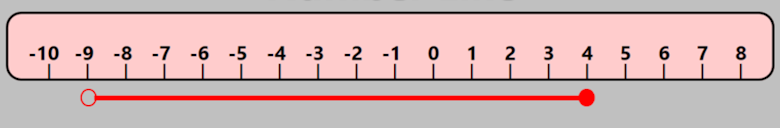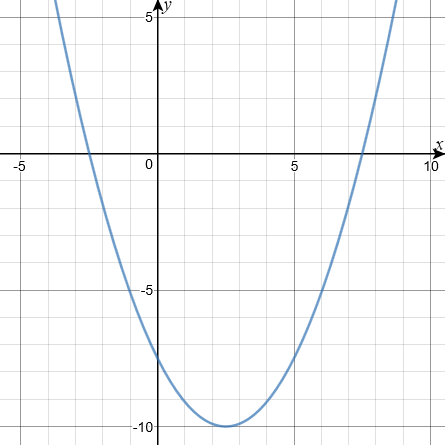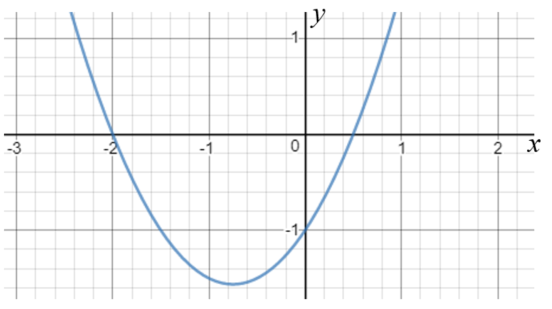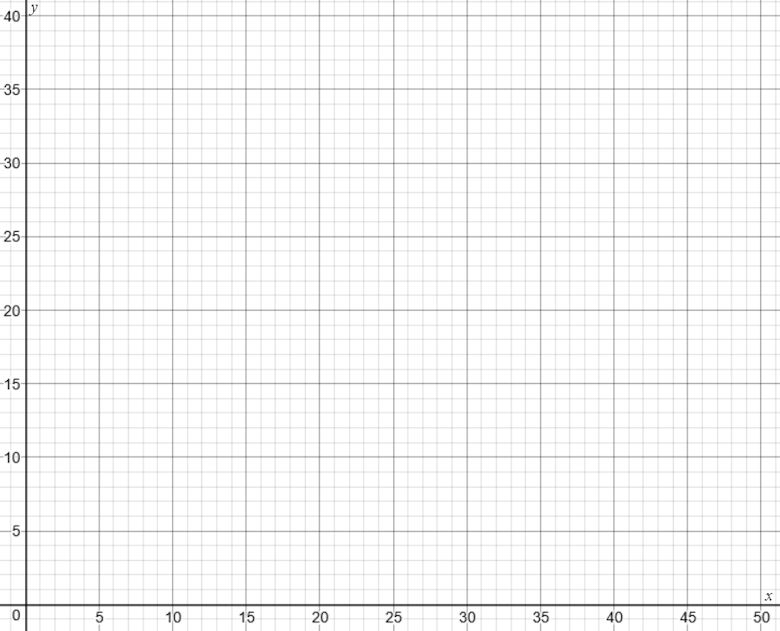# Exam-Style Questions.

## Problems adapted from questions set for previous Mathematics exams.

### 1.

GCSE Higher

The red line in the diagram below shows an inequality. If the variable is $$x$$, which inequality best describes $$x$$?(a) $$-9 \le x \le 4$$

(b) $$-9 \lt x \le 4$$

(c) $$-9 \le x \lt 4$$

(d) $$-9 \lt x \lt 4$$

(e) $$-4 \le x \le 9$$

### 2.

GCSE Higher

The graph of a quadratic function, $$y=f(x)$$ is shown drawn accurately in the following diagram. Write down all the integer solutions of $$f(x) \le 0$$.### 3.

GCSE Higher

The diagram below is a sketch of $$y = f(x)$$ where $$f(x)$$ is a quadratic function.

The graph intersects the x-axis where $$x=-2$$ and $$x = 0.5$$.Which of the following is the solution of $$f(x) \le 0$$ ?

• (a) $$x \ge -2$$ or $$x \ge 0.5$$

• (b) $$-2 \ge x \ge 0.5$$

• (c) $$x \ge -2$$ and $$x \le 0.5$$

• (d) $$x \le -2$$ and $$x \ge 0.5$$

### 4.

GCSE Higher

Describe the unshaded (white) region by writing down three inequalities.### 5.

GCSE Higher

On the grid below indicate the region that satisfies all three of these inequalities.

$$y>-2$$ $$x+y<5$$ $$y-1 \le \frac{x}{2}$$### 6.

GCSE Higher

A region on a coordinate grid is described by the following three inequalities:

$$x>-2$$ $$x+y<7$$ $$y \ge \frac{x}{3}+2$$

By shading the unwanted regions show the region on the grid below.### 7.

GCSE Higher

By shading the unwanted regions, show the region satisfied by these three inequalities.

$$y \le x + 3$$ $$y> 4-x$$ $$x < 2.5$$### 8.

GCSE Higher

Solve the following inequalities then explain how the whole number solutions to A and B different.

$$A: 5 \le 5x \lt 30$$ $$B: 5 \lt 5x \le 30$$

### 9.

IGCSE Extended

A large car park has an area of 1400m2 with space for $$x$$ cars and $$y$$ vans. Each car requires 14m2 of space and each van requires 35m2 of space.

(a) Show that $$2x+5y \le 200$$

(b) There must also be space for

(i) at least 50 vehicles,
(ii) at least 20 vans.

Write down two more inequalities to show this information.

(c) On the grid, show the three inequalities by drawing the three lines and shading the unwanted regions.(d) Use your graph to find the largest possible number of vans.

(e) The company charges £6 for parking each car and £10 for parking each van. Find the number of cars and the number of vans which give the owners of the car park the greatest possible income and calculate this income.

### 10.

GCSE Higher

Show that you understand equations and inequalities by answering the following:

(a) Solve $$5x^2=80$$

(b) Solve $$8x + 2 \gt x + 7$$

(c) Write down the largest integer that satisfies $$8x - 2 \lt 25$$

(d) Solve the following pair of equations

$$3x + 5y = 21$$ $$8x - 5y = 1$$

### 11.

GCSE Higher

Here is a function machine that produces two outputs, A and B.Work out the range of input values for which the output A is less than the output B.

### 12.

GCSE Higher

Solve $$2x^2 + 7x - 15 <0$$

The exam-style questions appearing on this site are based on those set in previous examinations (or sample assessment papers for future examinations) by the major examination boards. The wording, diagrams and figures used in these questions have been changed from the originals so that students can have fresh, relevant problem solving practice even if they have previously worked through the related exam paper.

The solutions to the questions on this website are only available to those who have a Transum Subscription.

Exam-Style Questions Main Page

Search for exam-style questions containing a particular word or phrase:

To search the entire Transum website use the search box in the grey area below.